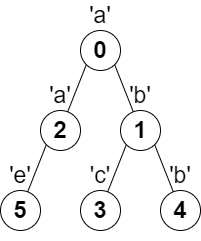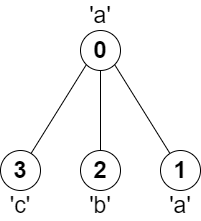2246. Longest Path With Different Adjacent Characters
Hard
2.2K
58

You are given a tree (i.e. a connected, undirected graph that has no cycles) rooted at node `0` consisting of `n` nodes numbered from `0` to `n - 1`. The tree is represented by a 0-indexed array `parent` of size `n`, where `parent[i]` is the parent of node `i`. Since node `0` is the root, `parent == -1`.

You are also given a string `s` of length `n`, where `s[i]` is the character assigned to node `i`.

Return the length of the longest path in the tree such that no pair of adjacent nodes on the path have the same character assigned to them.

Example 1:```Input: parent = [-1,0,0,1,1,2], s = "abacbe"
Output: 3
Explanation: The longest path where each two adjacent nodes have different characters in the tree is the path: 0 -> 1 -> 3. The length of this path is 3, so 3 is returned.
It can be proven that there is no longer path that satisfies the conditions.
```

Example 2:```Input: parent = [-1,0,0,0], s = "aabc"
Output: 3
Explanation: The longest path where each two adjacent nodes have different characters is the path: 2 -> 0 -> 3. The length of this path is 3, so 3 is returned.
```

Constraints:

• `n == parent.length == s.length`
• `1 <= n <= 105`
• `0 <= parent[i] <= n - 1` for all `i >= 1`
• `parent == -1`
• `parent` represents a valid tree.
• `s` consists of only lowercase English letters.
Accepted
65.4K
Submissions
117.1K
Acceptance Rate
55.8%

Seen this question in a real interview before?
1/4
Yes
No

Discussion (0)

Related Topics